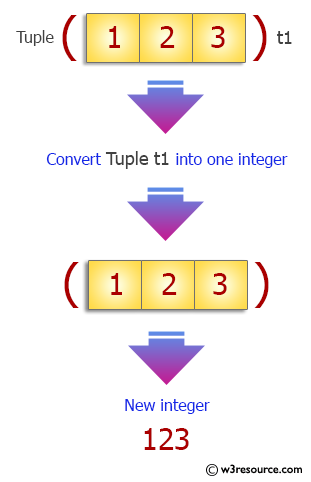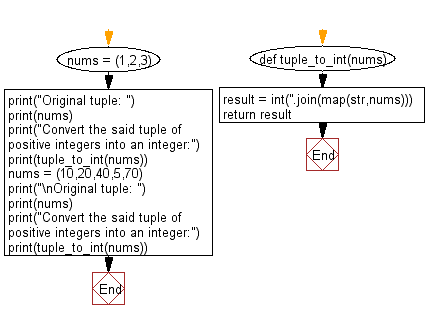﻿ Python: Convert a given tuple of positive integers into an integer - w3resource# Python Exercise: Convert a given tuple of positive integers into an integer

## Python tuple: Exercise-29 with Solution

Write a Python program to convert a given tuple of positive integers into an integer.

Sample Solution:-

Python Code:

``````def tuple_to_int(nums):
result = int(''.join(map(str,nums)))
return result

nums = (1,2,3)
print("Original tuple: ")
print(nums)
print("Convert the said tuple of positive integers into an integer:")
print(tuple_to_int(nums))

nums = (10,20,40,5,70)
print("\nOriginal tuple: ")
print(nums)
print("Convert the said tuple of positive integers into an integer:")
print(tuple_to_int(nums))
```
```

Sample Output:

```Original tuple:
(1, 2, 3)
Convert the said tuple of positive integers into an integer:
123

Original tuple:
(10, 20, 40, 5, 70)
Convert the said tuple of positive integers into an integer:
102040570
```

Pictorial Presentation:Flowchart:## Visualize Python code execution:

The following tool visualize what the computer is doing step-by-step as it executes the said program:

Python Code Editor:

Have another way to solve this solution? Contribute your code (and comments) through Disqus.

What is the difficulty level of this exercise?

Test your Programming skills with w3resource's quiz.

﻿

## Python: Tips of the Day

Decapitalizes the first letter of a string:

Example:

```def tips_decapitalize(s, upper_rest=False):
return s[:1].lower() + (s[1:].upper() if upper_rest else s[1:])
print(tips_decapitalize('PythonTips'))
print(tips_decapitalize('PythonTips', True))
```

Output:

```pythonTips
pYTHONTIPS
```